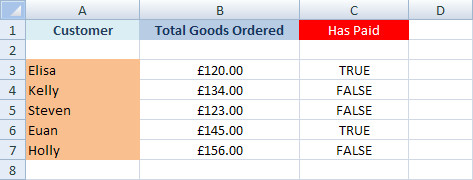# The Excel SumIF Function

Another useful Excel function is SumIF. This function is like CountIf, except it adds one more argument:

SUMIF(range, criteria, sum_range)

Range and criteria are the same as with CountIF - the range of cells to search, and what you want Excel to look for. The Sum_Range is like range, but it searches a new range of cells. To clarify all that, here's what we'll use SumIF for. (Start a new spreadsheet for this.)

Five people have ordered goods from us. Some have paid us, but some haven't. The five people are Elisa, Kelly, Steven, Euan, and Holly. We'll use SumIF to calculate how much in total has been paid to us, and how much is still owed.

So in Column A, enter the names:In Column B enter how much each person owes:In Column C, enter TRUE or FALSE values. TRUE means they have paid up, and FALSE means they haven't:Add two more labels: Total Paid, and Still Owed. Your spreadsheet should look something like this one:In cells B10 and B11, we'll use a SumIF function to work out how much has been paid in, and how much is still owed. Here's the SumIF function again:

SUMIF(range, criteria, sum_range)

So the range of cells that we want to check are the True and False values in the C column; the criteria is whether they have paid (True); and the Sum_Range is what we want to add up (in the B column).

In cell B10, then, enter the following formula:

=SUMIF(C3:C7, TRUE, B3:B7)

When you press the enter key, Excel should give you the answer:So 265 is has been paid in. But we told SumIF to first check the values in the cells C3 to C7 (range). Then we said look for a value of TRUE (criteria). Finally, we wanted the values in the B column adding up, if a criteria of TRUE was indeed found (sum_range).

Exercise
Use SumIF to work out how much is still owed. Put your answer in cell B11.

We'll leave the subject of Conditional Logic. In the next section, more things you can do with Excel!

<--Back to the Excel Contents Page